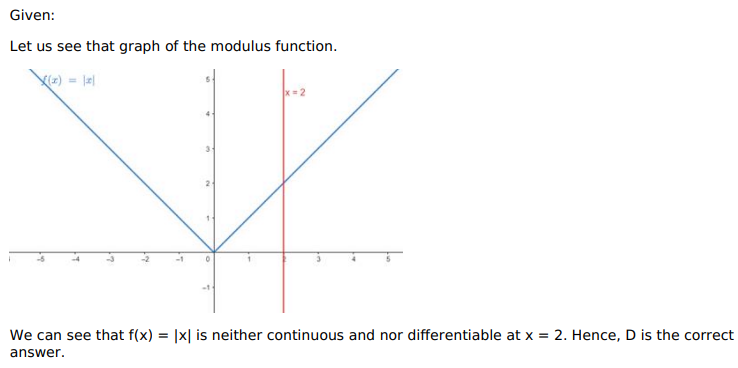# Solve this following

Question:

Mark $(\sqrt{)}$ against the correct answer in the following:

At $\mathrm{x}=2, \quad f(\mathrm{x})=|\mathrm{x}|$ is

A. continuous but not differentiable

B. differentiable but not continuous

C. continuous as well as differentiable

D. none of these

Solution: